Mathematics: CUET Mock Test - 6

# Mathematics: CUET Mock Test - 6

Test Description

## 40 Questions MCQ Test CUET Science Subjects Mock Tests | Mathematics: CUET Mock Test - 6

Mathematics: CUET Mock Test - 6 for Class 12 2023 is part of CUET Science Subjects Mock Tests preparation. The Mathematics: CUET Mock Test - 6 questions and answers have been prepared according to the Class 12 exam syllabus.The Mathematics: CUET Mock Test - 6 MCQs are made for Class 12 2023 Exam. Find important definitions, questions, notes, meanings, examples, exercises, MCQs and online tests for Mathematics: CUET Mock Test - 6 below.
Solutions of Mathematics: CUET Mock Test - 6 questions in English are available as part of our CUET Science Subjects Mock Tests for Class 12 & Mathematics: CUET Mock Test - 6 solutions in Hindi for CUET Science Subjects Mock Tests course. Download more important topics, notes, lectures and mock test series for Class 12 Exam by signing up for free. Attempt Mathematics: CUET Mock Test - 6 | 40 questions in 45 minutes | Mock test for Class 12 preparation | Free important questions MCQ to study CUET Science Subjects Mock Tests for Class 12 Exam | Download free PDF with solutions
 1 Crore+ students have signed up on EduRev. Have you?
Mathematics: CUET Mock Test - 6 - Question 1

### Which of these is not a type of relation?

Detailed Solution for Mathematics: CUET Mock Test - 6 - Question 1

Surjective is not a type of relation. It is a type of function. Reflexive, Symmetric and Transitive are type of relations.

Mathematics: CUET Mock Test - 6 - Question 2

### What will be the value of x + y + z if cos-1 x + cos-1 y + cos-1 z = 3π?

Detailed Solution for Mathematics: CUET Mock Test - 6 - Question 2

The equation is cos-1 x + cos-1 y + cos-1 z = 3π
This means cos-1 x = π, cos-1 y = π and cos-1 z = π
This will be only possible when it is in maxima.
As, cos-1 x = π so, x = cos-1 π = -1 similarly, y = z = -1
Therefore, x + y + z = -1 -1 -1
So, x + y + z = -3.

Mathematics: CUET Mock Test - 6 - Question 3

### If the order of the matrix is m×n, then how many elements will there be in the matrix?

Detailed Solution for Mathematics: CUET Mock Test - 6 - Question 3

The number of elements for a matrix with the order m×n is equal to mn, where m is the number of rows and n is the number of columns in the matrix.

Mathematics: CUET Mock Test - 6 - Question 4

The matrix which follows the conditions m=n is called?

Detailed Solution for Mathematics: CUET Mock Test - 6 - Question 4

A square matrix is a matrix in which the number of rows(m) is equal to the number of columns(n). Therefore, the matrix which follows the condition m = n is a square matrix.

Mathematics: CUET Mock Test - 6 - Question 5

Evaluate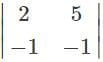.

Detailed Solution for Mathematics: CUET Mock Test - 6 - Question 5

Expanding along R1, we get
∆=2(-1)-5(-1)=-2+5
= 3.

Mathematics: CUET Mock Test - 6 - Question 6

Which of the following matrices will not have a determinant?

Detailed Solution for Mathematics: CUET Mock Test - 6 - Question 6

Determinant of the matrix A=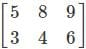is not possible as it is a rectangular matrix and not a square matrix. Determinants can be calculated only if the matrix is a square matrix.

Mathematics: CUET Mock Test - 6 - Question 7

Which value is similar to sin-1sin(6 π/7)?

Detailed Solution for Mathematics: CUET Mock Test - 6 - Question 7

sin-1sin(6 π/7)
Now, sin(6 π/7) = sin(π – 6 π/7)
= sin(2π + 6 π/7) = sin(π/7)
= sin(3π – 6 π/7) = sin(20π/7)
= sin(-π – 6 π/7) = sin(-15π/7)
= sin(-2π + 6 π/7) = sin(-8π/7)
= sin(-3π – 6 π/7) = sin(-27π/7)
Therefore, sin-1sin(6 π/7) = sin-1(π/7).

Mathematics: CUET Mock Test - 6 - Question 8

Which of the following is a matrix of the order 2×2 where the equation of the elements is given by aij =i+j.

Detailed Solution for Mathematics: CUET Mock Test - 6 - Question 8

a11=1+1=2, a12=1+2=3, a21=2+1=3, a22=2+2=4
∴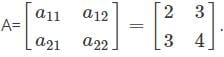Mathematics: CUET Mock Test - 6 - Question 9

Consider the matrix A=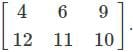What is the type of matrix?

Detailed Solution for Mathematics: CUET Mock Test - 6 - Question 9

The matrix in which number of rows is smaller than the number of columns is called is called a horizontal matrix. In the given matrix A=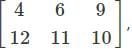m = 3 and n = 2 i.e.
3<2. Hence, it is a horizontal matrix.

Mathematics: CUET Mock Test - 6 - Question 10

Evaluate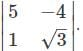Detailed Solution for Mathematics: CUET Mock Test - 6 - Question 10

Evaluating along R1, we get
∆ = 5(√3)-(-4)1 = 5√3+4.

Mathematics: CUET Mock Test - 6 - Question 11

Which of the following relations is symmetric but neither reflexive nor transitive for a set A = {1, 2, 3}.

Detailed Solution for Mathematics: CUET Mock Test - 6 - Question 11

A relation in a set A is said to be symmetric if (a1, a2)∈R implies that (a1, a2)∈R,for every a1, a2∈R.
Hence, for the given set A={1, 2, 3}, R={(1, 2), (2, 1)} is symmetric. It is not reflexive since every element is not related to itself and neither transitive as it does not satisfy the condition that for a given relation R in a set A if (a1, a2)∈R and (a2, a3)∈R implies that (a1, a3)∈ R for every a1, a2, a3∈R.

Mathematics: CUET Mock Test - 6 - Question 12

What is the value of sin-1(-x) for all x belongs to [-1, 1]?

Detailed Solution for Mathematics: CUET Mock Test - 6 - Question 12

Let, θ = sin-1(-x)
So, -π/2 ≤ θ ≤ π/2
⇒ -x = sinθ
⇒ x = -sinθ
⇒ x = sin(-θ)
Also, -π/2 ≤ -θ ≤ π/2
⇒ -θ = sin-1(x)
⇒ θ = -sin-1(x)
So, sin-1(-x) = -sin-1(x)

Mathematics: CUET Mock Test - 6 - Question 13

What is the order of the matrix A =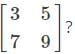Detailed Solution for Mathematics: CUET Mock Test - 6 - Question 13

The number of rows (m) and the number of columns (n) in the given matrix A=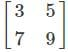is 2. Therefore, the order of the matrix is 2×2(m×n).

Mathematics: CUET Mock Test - 6 - Question 14

The matrix A=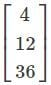is ____

Detailed Solution for Mathematics: CUET Mock Test - 6 - Question 14

The given matrix A =is of the order 3×1. The matrix has only one column (n=1). Hence, it is a column matrix.

Mathematics: CUET Mock Test - 6 - Question 15

Evaluate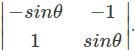Detailed Solution for Mathematics: CUET Mock Test - 6 - Question 15

Expanding along R1, we get
∆=-sinθ(sinθ)-(-1)1=-sin2⁡θ+1=cos2⁡θ.

Mathematics: CUET Mock Test - 6 - Question 16

Which of the following relations is transitive but not reflexive for the set S={3, 4, 6}?

Detailed Solution for Mathematics: CUET Mock Test - 6 - Question 16

For the above given set S = {3, 4, 6}, R = {(3, 4), (4, 6), (3, 6)} is transitive as (3, 4)∈R and (4, 6) ∈R and (3,6) also belongs to R . It is not a reflexive relation as it does not satisfy the condition (a, a) ∈ R, for every a ∈ A for a relation R in the set A.

Mathematics: CUET Mock Test - 6 - Question 17

What is the value of sin-1(sin 6)?

Detailed Solution for Mathematics: CUET Mock Test - 6 - Question 17

We know that sin(x) = sin(2A * π + x) where A can be positive or negative integer.
If A is -1, then sin(6) = sin(-2π + 6);
If A is 1, then sin(6) = sin(2π + 6);

Mathematics: CUET Mock Test - 6 - Question 18

What is the value of r = 1Σn f(x) if f(r) =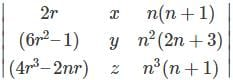where n € N?

Detailed Solution for Mathematics: CUET Mock Test - 6 - Question 18

The given determinant is f(r) =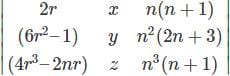Now, r = 1Σn (2r) = 2[(n(n + 1))/2] ……….(1)
= n2 + n
r = 1Σn(6r2 – 1) = 6[((n + 1)(2n + 1))/6] – n ……….(2)
= n(2n2 + 2n + n + 1) – n
= 2n3 + 2n2 + n2 + n – n
= 2n3 + 3n2
= r = 1Σn(4r3 – 2nr) = n3 (n + 1) ……….(3)
From (1), (2) and (3) we get
r = 1Σn f(x) = 0

Mathematics: CUET Mock Test - 6 - Question 19

The matrix which follows the condition m>n is called as _______

Detailed Solution for Mathematics: CUET Mock Test - 6 - Question 19

The matrix in which the number of columns is greater than the number of rows is called a vertical matrix. There the matrix which follows the condition m>n is a vertical matrix.

Mathematics: CUET Mock Test - 6 - Question 20

Evaluate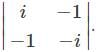Detailed Solution for Mathematics: CUET Mock Test - 6 - Question 20

Expanding along R1, we get
∆=-i(i)-(-1)(-1)=-i2-1=-(-1)-1=0.

Mathematics: CUET Mock Test - 6 - Question 21

Let R be a relation in the set N given by R={(a,b): a+b=5, b>1}. Which of the following will satisfy the given relation?

Detailed Solution for Mathematics: CUET Mock Test - 6 - Question 21

(2, 3) ∈ R as 2 + 3 = 5, 3 >1, thus satisfying the given condition.
(4, 2) doesn’t belong to R as 4 + 2 ≠ 5.
(2,1) doesn’t belong to R as 2+ 1 ≠ 5.
(5, 0) doesn’tbelong to R as 0 ⊁ 1

Mathematics: CUET Mock Test - 6 - Question 22

What is the value of cos-1(-x) for all x belongs to [-1, 1]?

Detailed Solution for Mathematics: CUET Mock Test - 6 - Question 22

Let, θ = cos-1(-x)
So, 0 ≤ θ ≤ π
⇒ -x = cosθ
⇒ x = -cosθ
⇒ x = cos(-θ)
Also, -π ≤ -θ ≤ 0
So, 0 ≤ π -θ ≤ π
⇒ -θ = cos-1(x)
⇒ θ = -cos-1(x)
So, cos-1(x) = π – θ
θ = π – cos-1(x)
⇒ cos-1(-x) = π – cos-1(x)

Mathematics: CUET Mock Test - 6 - Question 23

Which one is correct, the following system of linear equations 2x – 3y + 4z = 7, 3x – 4y + 5z = 8, 4x – 5y + 6z = 9 has?

Detailed Solution for Mathematics: CUET Mock Test - 6 - Question 23

Solving the given system of equation by Cramer’s rule, we get,
x = D1/D, y = D2/D, z = D3/D where,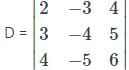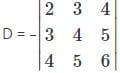Now, performing, C3 = C3 – C2 and C2 = C2 – C1 we get,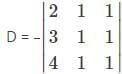As two columns have identical values, so,
D = 0
Similarly,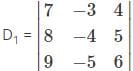Now, performing, C1 = C1 – C3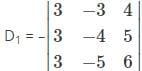Now, performing, C3 = C3 – C2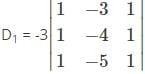As two columns have identical values, so,
D1 = 0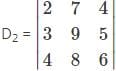Now, performing,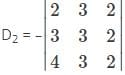Now, performing, C2 = C2 – C3 and C3 = C3 – C1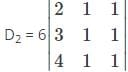As two columns have identical values, so,
D2 = 0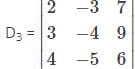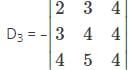Now, performing, C2 = C2 – C2 and C3 = C3 – C2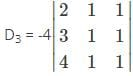As two columns have identical values, so,
D3 = 0
Since, D = D1 = D2 = D3 = 0, thus, it has infinitely many solutions.

Mathematics: CUET Mock Test - 6 - Question 24

Find the value of a,b,c,d if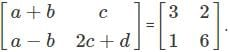Detailed Solution for Mathematics: CUET Mock Test - 6 - Question 24

The two matrices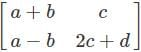and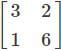are equal matrices. Comparing the two matrices, we get a-b=3, c=2, a-b=1, 2c+d=6
Solving the above equations, we get a=2, b=1, c=2, d=2.

Mathematics: CUET Mock Test - 6 - Question 25

Evaluate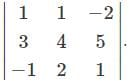Detailed Solution for Mathematics: CUET Mock Test - 6 - Question 25

∆=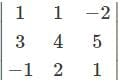Expanding along the first row, we get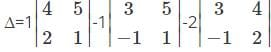=1(4-5(2))-1(3-5(-1))-2(6-4(-1))
=(4-10)-(3+5)-2(6+4)
=-6-8-20=-34.

Mathematics: CUET Mock Test - 6 - Question 26

Which of the following relations is reflexive but not transitive for the set T = {7, 8, 9}?

Detailed Solution for Mathematics: CUET Mock Test - 6 - Question 26

The relation R= {(7, 7), (8, 8), (9, 9)} is reflexive as every element is related to itself i.e. (a, a) ∈ R, for every a∈A. and it is not transitive as it does not satisfy the condition that for a relation R in a set A if (a1, a2)∈R and (a2, a3)∈R implies that (a1, a3) ∈ R for every a1, a2, a3 ∈ R.

Mathematics: CUET Mock Test - 6 - Question 27

The given graph is for which equation?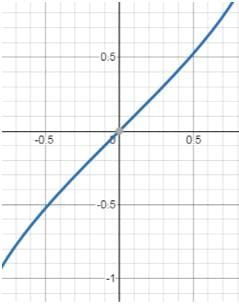Detailed Solution for Mathematics: CUET Mock Test - 6 - Question 27

The following graph represents 2 equations.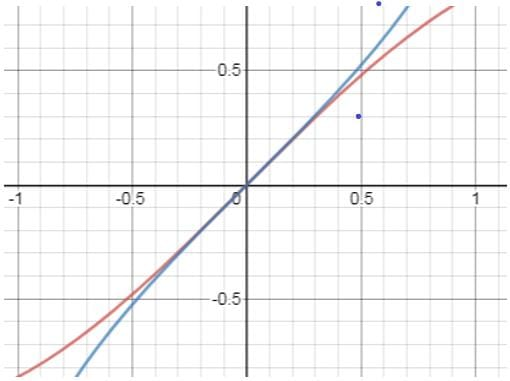The pink curve is the graph of y = sinx
The blue curve is the graph for y = sin-1x
This curve passes through the origin and approaches to infinity in both positive and negative axes.

Mathematics: CUET Mock Test - 6 - Question 28

Which of the following is not a possible ordered pair for a matrix with 6 elements.

Detailed Solution for Mathematics: CUET Mock Test - 6 - Question 28

The possible orders in which the matrix with 6 elements can be formed are 2×3, 3×2, 1×6, 6×1. Therefore, the possible orders pairs are (2,3), (3,2), (1,6), (6,1). Thus, (3,1) is not possible.

Mathematics: CUET Mock Test - 6 - Question 29

Which of the following is a diagonal matrix.

Detailed Solution for Mathematics: CUET Mock Test - 6 - Question 29

The matrix is said to be a diagonal matrix if the elements along the diagonal of the matrix are non – zero.
i.e. aij=0 for i≠j and aij≠0 for i=j.
Therefore, the matrix A=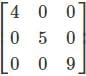is a diagonal matrix.

Mathematics: CUET Mock Test - 6 - Question 30

Evaluate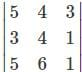.

Detailed Solution for Mathematics: CUET Mock Test - 6 - Question 30

Expanding along the first row, we get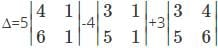=5(4-6)-4(3-5)+3(18-20)
=5(-2)-4(-2)+3(-2)=-10+8-6=-8.

Mathematics: CUET Mock Test - 6 - Question 31

Let I be a set of all lines in a XY plane and R be a relation in I defined as R = {(I1, I2):I1 is parallel to I2}. What is the type of given relation?

Detailed Solution for Mathematics: CUET Mock Test - 6 - Question 31

This is an equivalence relation. A relation R is said to be an equivalence relation when it is reflexive, transitive and symmetric.
Reflexive: We know that a line is always parallel to itself. This implies that I1 is parallel to I1 i.e. (I1, I2)∈R. Hence, it is a reflexive relation.
Symmetric: Now if a line I1 || I2 then the line I2 || I1. Therefore, (I1, I2)∈R implies that (I2, I1)∈R. Hence, it is a symmetric relation.
Transitive: If two lines (I1, I3) are parallel to a third line (I2) then they will be parallel to each other i.e. if (I1, I2) ∈R and (I2, I3) ∈R implies that (I1, I3) ∈R.

Mathematics: CUET Mock Test - 6 - Question 32

The given graph is for which equation?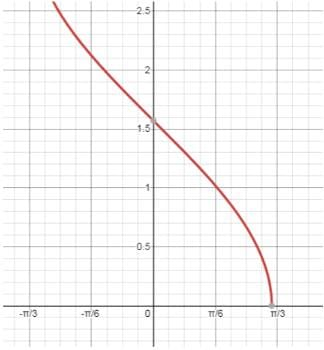Detailed Solution for Mathematics: CUET Mock Test - 6 - Question 32

There are 2 curves.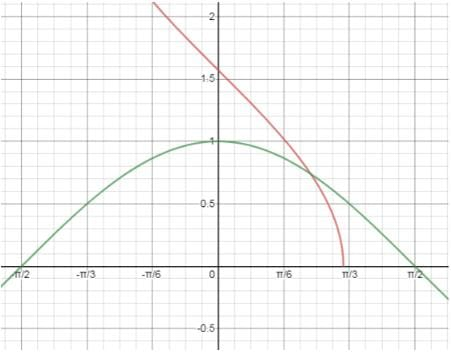The green curve is the graph of y = cosx
The red curve is the graph for y = cos-1x
This curve origin from some point before π/3 and approaches to infinity in both positive y axis by intersecting at a point near 1.5 in y axis.

Mathematics: CUET Mock Test - 6 - Question 33

Which of the following matrix is of the order 3×4.

Detailed Solution for Mathematics: CUET Mock Test - 6 - Question 33

The matrix A=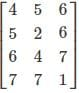is a 3×4 matrix as it as 3 rows and 4 columns.

Mathematics: CUET Mock Test - 6 - Question 34

Find the value of k if the area is 7/2 sq. units and the vertices are (1,2), (3,5), (k,0).

Detailed Solution for Mathematics: CUET Mock Test - 6 - Question 34

Given that the vertices are (1,2), (3,5), (k,0)
Therefore, the area of the triangle with vertices (1,2), (3,5), (k,0) is given by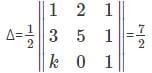Expanding along R3, we get
(1/2){k(2-5)-0+1(5-6)}=(1/2){-3k-1}=(7/2)
⇒ -(1/2)(3k+1)=7/2
3k=-8
k = -(8/3)

Mathematics: CUET Mock Test - 6 - Question 35

Evaluate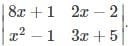Detailed Solution for Mathematics: CUET Mock Test - 6 - Question 35

Expanding along the first row, we get
∆=8x+1(3x+5)-(2x-2)(x2-1)
=(24x2+43x+5)-(2x3-2x2-2x+2)
=-2x3+26x2+45x+3.

Mathematics: CUET Mock Test - 6 - Question 36

Which of the following relations is symmetric and transitive but not reflexive for the set I = {4, 5}?

Detailed Solution for Mathematics: CUET Mock Test - 6 - Question 36

R= {(4, 5), (5, 4), (4, 4)} is symmetric since (4, 5) and (5, 4) are converse of each other thus satisfying the condition for a symmetric relation and it is transitive as (4, 5)∈R and (5, 4)∈R implies that (4, 4) ∈R. It is not reflexive as every element in the set I is not related to itself.

Mathematics: CUET Mock Test - 6 - Question 37

The given graph is for which equation?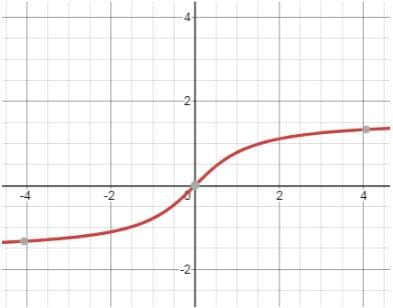Detailed Solution for Mathematics: CUET Mock Test - 6 - Question 37

There are 2 curves.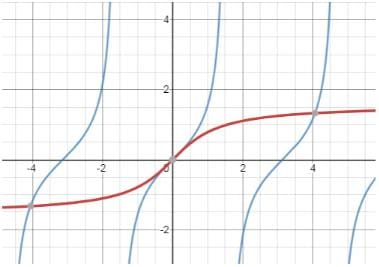The blue curve is the graph of y = tanx
The red curve is the graph for y = tan-1x
This curve passes through the origin and approaches to infinity in the direction of x axis only.
This graph lies below –x axis and above +x axis.

Mathematics: CUET Mock Test - 6 - Question 38

Consider the matrix A=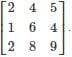. Find the element a32.

Detailed Solution for Mathematics: CUET Mock Test - 6 - Question 38

a32 is the element represented in the form aij i is the row number and j is the column number. Therefore, the element a32 is the element in the third row (i=3)and second column (j=2) which is 8.

Mathematics: CUET Mock Test - 6 - Question 39

Which of the following is a scalar matrix?

Detailed Solution for Mathematics: CUET Mock Test - 6 - Question 39

A matrix is called a scalar matrix if the elements along the diagonal of the matrix are equal and are non-zero i.e. aij=k for i=j and aij=0 for i≠j.
Therefore, the matrix A=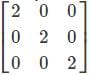is a scalar matrix.

Mathematics: CUET Mock Test - 6 - Question 40

If A=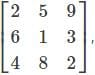find |A|.

Detailed Solution for Mathematics: CUET Mock Test - 6 - Question 40

Given that, A=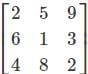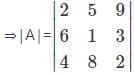Evaluating along the first row, we get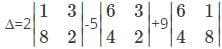∆=2(2-24)-5(12-12)+9(48-4)
∆=2(-22)-0+9(44)
∆=-44+9(44)=44(-1+9)=352

## CUET Science Subjects Mock Tests

60 tests
 Use Code STAYHOME200 and get INR 200 additional OFF Use Coupon Code
Information about Mathematics: CUET Mock Test - 6 Page
In this test you can find the Exam questions for Mathematics: CUET Mock Test - 6 solved & explained in the simplest way possible. Besides giving Questions and answers for Mathematics: CUET Mock Test - 6, EduRev gives you an ample number of Online tests for practice

60 tests